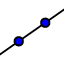# Circumcenter Construction

## Explore the construction of the circumcenter and its relationship with the triangle.

Summarize the properties and construction of the circumcenter of a triangle. Hint: If you are not familiar with the construction steps necessary, you might want to explore the applet below. Just use the buttons of the Navigation Bar in order to replay the construction steps.

Construct the circumcenter of triangle ABC by following the construction steps provided below the blank worksheet.

## Construction Steps:

 1. ﻿﻿Construct a circle centered at point A and through point B.﻿ (circle d) 8.﻿ ﻿﻿Construct line GF.﻿ (line i) ﻿2.Construct a circle centered at point B and through point A.﻿ (circle e)﻿ ﻿9. ﻿Construct a circle centered at point B and through point C.﻿ (circle k) ﻿﻿3. ﻿﻿Construct the intersection points between circle d and circle e. (point D & point E) ﻿10. ﻿﻿Construct a circle centered at point C and through point B.﻿ (circle p) ﻿4. ﻿﻿Construct line DE.﻿ (line f) 11. ﻿Construct the intersection points between circle k and circle p. (point I & point H) ﻿5. ﻿﻿Construct a circle centered at point A and through point C.﻿ (circle g) ﻿12 ﻿﻿Construct line IH.﻿ (line J)
 ﻿6. ﻿Construct a circle centered at point C and through point A.﻿ (circle h) 13. ﻿Construct the intersection of line f and line i. (point J) ﻿7. ﻿Construct the intersection points between circle g and circle h. (point G & point F) ﻿ ﻿ ﻿﻿

## Question:

Are line DE, line GF, and line IH always concurrent? (Move points A, B, and C to change the triangle and check your answer.)

Check all that apply